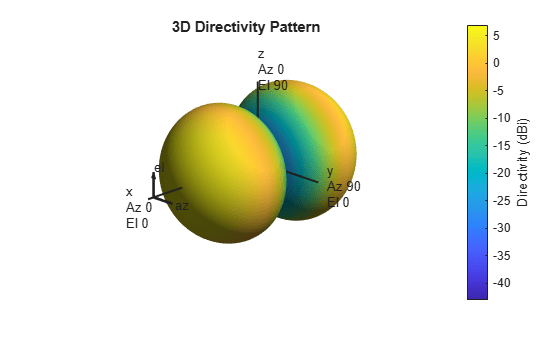# azelcut2pat

Create 3-D response pattern from azimuth and elevation cuts

Since R2019a

## Syntax

``pat = azelcut2pat(azcut,elcut)``

## Description

example

````pat = azelcut2pat(azcut,elcut)` creates a 3-D element response pattern, `pat`, from an azimuth cut, `azcut`, and an elevation cut, `elcut`. An azimuth cut consists of an antenna pattern over all azimuth angles at 0° elevation. An elevation cut consists of the antenna pattern over all elevation angles at 0° azimuth. You can specify cuts for different frequencies at the same time.```

## Examples

collapse all

Create a custom antenna pattern from azimuth and elevation cuts of a cosine-squared pattern.

```az = -180:180; azcut = mag2db(cosd(az).^2); el = -90:90; elcut = mag2db(cosd(el).^2); pat = azelcut2pat(azcut,elcut); antenna = phased.CustomAntennaElement('AzimuthAngles',az,... 'ElevationAngles',el,'MagnitudePattern',pat,... 'PhasePattern',zeros(size(pat)));```

Display the antenna pattern for 200 MHz.

```fs = 200.0e6; pattern(antenna,fs);```## Input Arguments

collapse all

Azimuth pattern cut, specified as a real-valued 1-by-Q vector or a real-valued L-by-Q matrix. Q is the number of azimuth angles, and L is the number of frequencies. Azimuth cuts are assumed to be made at 0° elevation. When `azcut` is a matrix, each column represents a different azimuth angle, and each row represents a different frequency. Units are in dB.

Data Types: `double`

Elevation pattern cut, specified as a real-valued 1-by-P vector or a real-valued L-by-P matrix. P is the number of elevation angles, and L is the number of frequencies. Elevation cuts are assumed to be made at 0° azimuth. When `elcut` is a matrix, each column represents a different elevation angle, and each row represents a different frequency. Units are in dB.

Data Types: `double`

## Output Arguments

collapse all

3-D array or antenna pattern, returned as a real-valued P-by-Q matrix or real-valued P-by-Q-by-L MATLAB® array. Units are in dB.

## Algorithms

The function returns a 3-D antenna pattern at the same azimuth and elevation angles used to define the `azcut` and `elcut` cuts. Because the cuts are specified in dB, the 3-D pattern is computed from the sum of the cut patterns.

pat(az,el) = pat(az) + pat(el)

## Version History

Introduced in R2019a